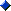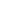Office Applications and Entertainment, Magic SquaresIndex About the Author

6.6   Medjig Solutions (Main Diagonals, Integers 0, 1, 2 and 3)

6.6.1 General

This method is based on a mathematical game called Medjig (author: Willem Barink, editor: Philos-Spiele, published: 2006).

The pieces of the Medjig Puzzle are squares divided in four quadrants on which the numbers 0, 1, 2 and 3 are dotted in all sequences.

There are 18 squares, with each sequence occurring 3 times.

The aim of the puzzle is to take 9 squares out of the collection and arrange them in a 3 x 3 "Medjig-square" in such a way that each row and column formed by the quadrants sums to 9, along with the two main diagonals.

For the sake of numerical analysis the Medjig pieces can be represented as follows:

 0 1 2 3
 0 1 3 2
 0 2 1 3
 0 2 3 1
 0 3 1 2
 0 3 2 1

*

 1 0 2 3
 1 0 3 2
 1 2 0 3
 1 2 3 0
 1 3 0 2
 1 3 2 0

*

 2 0 1 3
 2 0 3 1
 2 1 0 3
 2 1 3 0
 2 3 0 1
 2 3 1 0

*

 3 0 1 2
 3 0 2 1
 3 1 0 2
 3 1 2 0
 3 2 0 1
 3 2 1 0

The Medjig method of constructing a Magic Square of order 6 is as follows:Construct any 3 x 3 Medjig-Square A (ignoring the original game's limit on the number of times that a givensequence is used);Construct a 3 x 3 Magic Square B (only 8 possibilities);Construct a 6 x 6 Magic Square C by applying the equations shown below.

B (3 x 3)

 b1 b2 b3 b4 b5 b6 b7 b8 b9

Medjig Square A (3 x 3)

 a1 a2 a3 a4 a5 a6 a7 a8 a9 a10 a11 a12 a13 a14 a15 a16 a17 a18 a19 a20 a21 a22 a23 a24 a25 a26 a27 a28 a29 a30 a31 a32 a33 a34 a35 a36

Magic Square C (6 x 6)

 b1+9*a1 b1+9*a2 b2+9*a3 b2+9*a4 b3+9*a5 b3+9*a6 b1+9*a7 b1+9*a8 b2+9*a9 b2+9*a10 b3+9*a11 b3+9*a12 b4+9*a13 b4+9*a14 b5+9*a15 b5+9*a16 b6+9*a17 b6+9*a18 b4+9*a19 b4+9*a20 b5+9*a21 b5+9*a22 b6+9*a23 b6+9*a24 b7+9*a25 b7+9*a26 b8+9*a27 b8+9*a28 b9+9*a29 b9+9*a30 b7+9*a31 b7+9*a32 b8+9*a33 b8+9*a34 b9+9*a35 b9+9*a36

The rows, columns and main diagonals of Square C sum to 2 times the corresponding sum of Magic Square B plus 9 times the corresponding sum of Medjig square A which results in s1 = 2 * 15 + 9 * 9 = 111.

Further it is obvious that as b(i) ≠ b(j) for i ≠ j with i, j = 1, 2, ... 9 also c(m) ≠ c(n) for n ≠ m with n, m = 1, 2, ... 36.

A numerical example is shown below:

B (3 x 3)

 8 1 6 3 5 7 4 9 2

Medjig Square A (3 x 3)

 2 3 0 2 0 2 1 0 3 1 3 1 3 1 1 2 2 0 0 2 0 3 3 1 3 2 2 0 0 2 0 1 3 1 1 3

Magic Square C (6 x 6)

 26 35 1 19 6 24 17 8 28 10 33 15 30 12 14 23 25 7 3 21 5 32 34 16 31 22 27 9 2 20 4 13 36 18 11 29

Similarly, for any larger integer n, a magic square C of order 2n can be constructed from any n x n medjig-square
A with each row, column, and main diagonal summing to 3n, and any n x n magic square B, by applying the following equations: bi + n2 aj, with i = 1, 2, ... n2 and j = 1, 2, ... 4n2.

6.6.2 Further Analysis

Medjig Squares are described by the same set of linear equations as shown in section 6.2 for Magic Squares, however with magic sum 9 and following additional equations:

a( 1) + a( 2) + a( 7) + a( 8) = 6
a( 3) + a( 4) + a( 9) + a(10) = 6
a( 5) + a( 6) + a(11) + a(12) = 6
a(13) + a(14) + a(19) + a(20) = 6
a(15) + a(16) + a(21) + a(22) = 6
a(17) + a(18) + a(23) + a(24) = 6
a(25) + a(26) + a(31) + a(32) = 6
a(27) + a(28) + a(33) + a(34) = 6
a(29) + a(30) + a(35) + a(36) = 6

which can be reduced, by means of row and column manipulations, to the minimum number of linear equations:

a(31) =  9 - a(32) - a(33) - a(34) - a(35) - a(36)
a(29) =  6 - a(30) - a(35) - a(36)
a(27) =  6 - a(28) - a(33) - a(34)
a(25) =  6 - a(26) - a(31) - a(32)
a(19) =  9 - a(20) - a(21) - a(22) - a(23) - a(24)
a(17) =  6 - a(18) - a(23) - a(24)
a(15) =  6 - a(16) - a(21) - a(22)
a(13) =  6 - a(14) - a(19) - a(20)
a(11) =  6 + a(12) - a(16) + a(18) - a(21) + a(24) - a(26) - a(29) - a(31) - a(35)
a( 8) =  9 - 0.5*a(9) - 0.5*a(10) - a(12) - 0.5*a(14) + a(16) - 0.5*a(18) - 0.5*a(20) + a(21) - 0.5*a(24) +
- a(25)- a(26) - 0.5*a(27) - 0.5*a(28) - a(32) + a(35)
a( 7) =      a( 8) + a(14) - a(16) + a(20) - a(21) - a(25) + a(29) - a(31) + a(36)
a( 6) =  3 - a(12) - a(18) - a(24) + a(29) + a(35)
a( 5) = -3 - a(12) + a(16) + a(21) + a(26) + a(31)
a( 4) =  9 - a(10) - a(16) - a(22) - a(28) - a(34)
a( 3) = -3 - a( 9) + a(16) + a(22) + a(28) + a(34)
a( 2) =  3 - a( 8) - a(14) - a(20) + a(25) + a(31)
a( 1) = -3 + a( 2) + a(14) + a(16) + a(20) + a(21) + a(26) + a(27) + a(28) - a(29) - a(31) - a(35) - 2*a(36)

The linear equations shown above are ready to be solved, for the magic constant 9.

The solutions can be obtained by guessing a(9), a(10), a(12), a(14), a(16), a(18), a(20) ... a(24), a(26), a(28), a(30) and a(32) ... a(36) and filling out these guesses in the abovementioned equations.

To obtain the integers 0, 1, 2 and 3 also following relations should be applied:

0 =< a(i) =< 3        for i = 1, 2, ... 8, 11, 13, 15, 17, 19, 25, 27, 29, 31
Int(a(i)) = a(i)      for i = 8

which can be incorporated in a guessing routine, which can be used to generate a defined number of Medjig Squares within a reasonable time.

With the third row of Medjig pieces constant, an optimized guessing routine (MgcSqr6d) produced 808 Medjig Squares within 28 seconds, which are shown in Attachment 6.8.1.

The resulting Magic Squares, based on the 8 possible Magic Squares of the 3th order, are shown in Attachment 6.8.2.

The total number of possible 3 x 3 Medjig Squares for which the rows, columns and main diagonals sum to 9 is
1.740.800.

It should be noted that, although much faster, not all Magic Squares of the 6th order can be found by means of the Medjig Solution.

6.6.3 Symmetrical Main Diagonals

With the option 'Symmetrical Main Diagonals' enabled, routine MgcSqr6d counted 125184 Medjig Squares within 5,5 minutes, of which the first 120 are shown in Attachment 6.8.3.

A numerical example is shown below:

B (3 x 3)
 8 1 6 3 5 7 4 9 2
Medjig Square A (3 x 3)
 3 2 0 3 1 0 1 0 2 1 3 2 1 3 0 1 1 3 0 2 2 3 0 2 1 0 2 1 3 2 3 2 3 0 1 0
Magic Square C (6 x 6)
 35 26 1 28 15 6 17 8 19 10 33 24 12 30 5 14 16 34 3 21 23 32 7 25 13 4 27 18 29 20 31 22 36 9 11 2

A few (120 ea) of the resulting Magic Squares with Symmetrical Main Diagonals, based on the first 3th order Magic Square listed in routine MgcSqr6d, are shown in Attachment 6.8.4.

6.6.4 Almost Associated (16 pairs)

With the option 'Almost Associated' enabled, routine MgcSqr6d counted 7296 Almost Associated Medjig Squares within 52 seconds, of which the first 120 are shown in Attachment 6.8.5.

A numerical example is shown below:

B (3 x 3)
 8 1 6 3 5 7 4 9 2
Medjig Square A (3 x 3)
 3 2 0 3 1 0 1 0 2 1 3 2 1 3 0 1 1 3 0 2 2 3 0 2 1 0 2 1 3 2 3 2 3 0 1 0
Magic Square C (6 x 6)
 35 26 1 28 15 6 17 8 19 10 33 24 12 30 5 14 16 34 3 21 23 32 7 25 13 4 27 18 29 20 31 22 36 9 11 2

A few (120 ea) of the resulting Almost Associated Magic Squares, based on the first 3th order Magic Square listed in routine MgcSqr6d, are shown in Attachment 6.8.6.